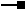# ITopologicalOperator3 Interface

Provides additional information on non-simple geometries.

## Members

Name DescriptionBoundary The boundary of this geometry. A polygon's boundary is a polyline. A polyline's boundary is a multipoint. A point or multipoint's boundary is an empty point or multipoint.Buffer Constructs a polygon that is the locus of points at a distance less than or equal to a specified distance from this geometry.Clip Constructs the intersection of this geometry and the specified envelope.ClipDense Constructs the intersection of this geometry and the specified envelope; densifies lines in output contributed by the clipping envelope.ClipToDomain Clips the geometry to the domain of the spatial reference. Useful for ensuring that buffers can be fit within the spatial domain of the feature class to which they are being added.ConstructBuffers Constructs a set of buffers at various distances. More efficient than calling Buffer repeatedly on the same geometry. This method is intended for internal use only.ConstructUnion Defines this geometry to be the union of the inputs. More efficient for unioning multiple geometries than calling Union repeatedly.ConvexHull Constructs the convex hull of this geometry.Cut Splits this geometry into a part left of the cutting polyline, and a part right of it.Difference Constructs the geometry containing points from this geometry but not the other geometry.GeoNormalizeEx Shifts longitudes, if need be, into a continuous range of 360 degrees.Intersect Constructs the geometry that is the set-theoretic intersection of the input geometries. Use different resultDimension values to generate results of different dimensions.IntersectMultidimension Constructs the set-theoretic intersection of the inputs. The results are returned in a geometry bag with one element per result dimension.IsKnownSimple Indicates whether this geometry is known (or assumed) to be topologically correct.IsKnownSimple Indicates whether this geometry is known (or assumed) to be topologically correct.IsSimple Indicates whether this geometry is known (or assumed) to be topologically correct, after explicitly determining this if the geometry is not already known (or assumed) to be simple.IsSimpleEx Determines why a geometry is not simple. Currently only implemented for polygons.QueryClipped Redefines clippedGeometry to be the intersection of this geometry and the clipping envelope.QueryClippedDense Redefines clippedGeometry to be the intersection of this geometry and the clipping envelope; densifies lines in the output contributed by the clipping envelope.Simplify Makes this geometry topologically correct.SymmetricDifference Constructs the geometry that contains points from either but not both input geometries.TopologyCache Provides a handle to the TopologyCache.Union Constructs the geometry that is the set-theoretic union of the input geometries.

### ITopologicalOperator3.GeoNormalizeEx Method

Shifts longitudes, if need be, into a continuous range of 360 degrees.

``````Public Sub GeoNormalizeEx ( _
ByVal SR As ISpatialReference, _
ByVal denseDistance As Double _
)
``````
``````public void GeoNormalizeEx (
ISpatialReference SR,
double denseDistance
);
``````

#### Remarks

Not implemented for Multipatches.

### ITopologicalOperator3.IsSimpleEx Property

Determines why a geometry is not simple. Currently only implemented for polygons.

``````Public Function get_IsSimpleEx ( _
ByRef reason As esriNonSimpleReasonEnum _
) As Boolean
``````
``````public bool get_IsSimpleEx (
ref esriNonSimpleReasonEnum reason
);
``````

#### Description

The return boolean value indicates whether or not the geometry is simple. If the return is false, the "reason" parameter can be inspected.

#### Remarks

Note: All geometries involved in ITopologicalOperator operations must be high-level geometries. High-Level geometries are point, multipoint, polyline and polygon. To use it with low-level geometries such as segments (Line, Circular Arc, Elliptic Arc, Bézier Curve), path or ring they must be wrapped into high-level geometries type.

The xy cluster tolerance value of the geometry's associated spatial reference is used by this method. If the goal of this method is to determine if a geometry can be persisted in an sde (or other integer-based) layer without alteration, you may wish to use the minimum xy cluster tolerance value (ISpatialReferenceTolerance::SetMinimumXYTolerance) before applying this method (don't forget to set it back).

``````//How to use get_IsSimpleEx

ITopologicalOperator3 topoOp3 = inGeom as ITopologicalOperator3; //Must be polygon

ESRI.ArcGIS.Geometry.esriNonSimpleReasonEnum reason = ESRI.ArcGIS.Geometry.esriNonSimpleReasonEnum.esriNonSimpleOK; //Initialize

topoOp3.IsKnownSimple_2 = false;

Boolean pBool = topoOp3.get_IsSimpleEx(out reason); //Inspect pReason for description code

//The following code shows to wrap a line segment into a polyline in C#

//Assume a line (line1 as ILine) is already created

object obj = Type.Missing;

ISegmentCollection segCollection = new PolylineClass() as ISegmentCollection;

//Set the spatial reference on the new polyline

//The spatial reference is not transfered automatically from the segments

IGeometry geom = segCollection as IGeometry;

geom.SpatialReference = spatialRef;

//Can now be used with ITopologicalOperator methods
``````

## Inherited Interfaces

Interfaces Description
ITopologicalOperator Provides access to members for constructing new geometries based upon topological relationships between existing geometries.

## Classes that implement ITopologicalOperator3

Classes Description
MultiPatch A collection of surface patches.
Polygon A collection of rings ordered by their containment relationship; optionally has measure, height and ID attributes.
Polyline An ordered collection of paths; optionally has measure, height and ID attributes.

## Remarks

ITopologicalOperator3 methods must be applied on high-level geometries only. High-Level geometries are point, multipoint, polyline and polygon. To use this interface with low-level geometries such as segments (Line, Circular Arc, Elliptic Arc, Bezier Curve), paths or rings, they must be wrapped into high-level geometry types.